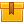# Flow Measurement (AFM02)

## Prerequisites

This lesson is designed for participants familiar with basic mathematical operations including algebra.

## Description

This lesson describes the properties of fluids that are a factor in the measurement of their flow. In addition, the lesson explains how differential pressure measurements can be used to determine flow rate. Various types of flow measurement devices and their principles of operation are also discussed.

## Objectives

Recognize the effect of temperature and pressure on the density and volume of a liquid and a gas. Describe the effects of temperature on viscosity and how viscosity affects flow. Describe laminar flow, turbulent flow, and transitional flow. Describe the application of the Reynolds Number to flow measurement. Identify variables that affect mass flow rate. Describe how static pressure is converted to kinetic energy. Explain Bernoulli??s law as it applies to differential pressure flow measurements. Explain the necessity of an expansion factor in differential pressure flow measurements of a gas. Describe how an orifice-type differential pressure flow device measures flow. Explain how a beta ratio is determined and its application to flow measurements. Identify the location of high and low pressure taps in an orifice run. Describe the design and operation of Venturi tube, flow nozzle, and Pitot tube differential pressure flow devices. Explain the difference between closed and open systems. Describe the design and operation of weir and flume head-type differential pressure flow devices. Describe the design and operation of vortex shedding, magnetic, ultrasonic, rotary vane, turbine, and Coriolis mass flowmeters. Describe the operational principles of positive displacement flowmeters. Describe how an inferential mass flow measurement differs from a true mass flow measurement.

## ContentThis lesson describes the properties of fluids that are a factor in the
measurement of their flow. In addition, the lesson explains how
differential pressure measurements can be used to determine flow rate.
Various types of flow measurement devices and their principles of
operation are also discussed.

•Flow Measurement

0
0 Reviews